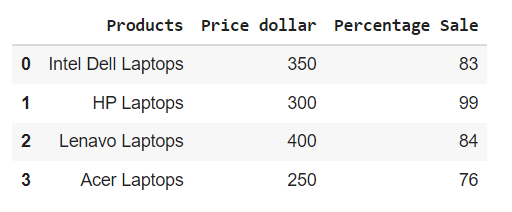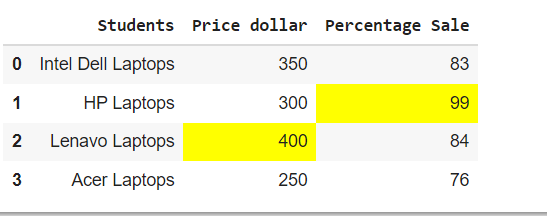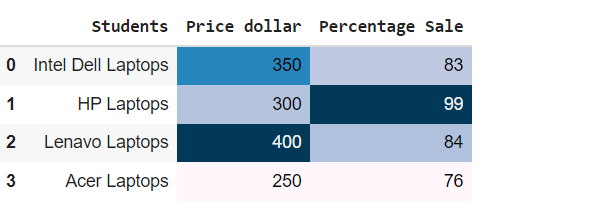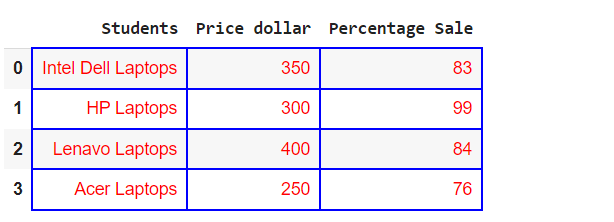# Pandas Display DataFrame in a Table Style

Samreena Aslam Jan 30, 2023 Oct 31, 2021 Pandas Pandas DataFrame

Pandas is a very popular and useful data science library. Today, every person that is involved in data science also uses the Pandas extensively. It displays the data in tabular form, which is quite similar to the format we see in the excel tool. Using the excel tool, we can customize our work or data sheets by adding various colors and styles that make them more attractive and readable for other users. Displaying pandas `DataFrame` via various table styles increases the data visualization.

We will introduce how to display the Pandas `DataFrame` in the form of tables using different table styles, such as the `tabulate` library, `dataframe.style`, and the `IPython.display` module.

## Display Pandas `DataFrame` in a Table by Using the `display()` Function of `IPython.display` Module

The simplest and easiest way to display pandas `DataFrame` in a table style is by using the `display()` function that imports from the `IPython.display` module. This function displays the `DataFrame` in an interactive and well-formatted tabular form.

See the following example for a good understanding of the `display()` function:

Example Codes:

``````from IPython.display import display
import pandas as pd

# creating a DataFrame
dict = {'Products' : ['Intel Dell Laptops', 'HP Laptops', 'Lenavo Laptops', 'Acer Laptops'],
'Price dollar' : [350, 300, 400, 250 ],
'Percentage Sale' : [83, 99, 84, 76]}
dataframe = pd.DataFrame(dict)

# displaying the DataFrame
display(dataframe)
``````

Output:## Display Pandas `DataFrame` in a Table Style Using the `tabulate` Library

Using the above method, we can display the pandas `dataframes` in an organized table style format. We will use a library known as `tabulate`. This library consists of different styles in which we can display pandas `dataframes`.

We will use the `pretty` style to display pandas `DataFrame` in the following example:

Example Codes:

``````import pandas as pd
from tabulate import tabulate

# creating a DataFrame
dict = {'Students' : ['Intel Dell Laptops', 'HP Laptops', 'Lenavo Laptops', 'Acer Laptops'],
'Price dollar' : [350, 300, 400, 250 ],
'Percentage Sale' : [83, 99, 84, 76]}
dataframe = pd.DataFrame(dict)

# displaying the DataFrame
print(tabulate(dataframe, headers = 'keys', tablefmt = 'pretty'))
``````

Output:

``````+---+--------------------+--------------+-----------------+
|   |      Students      | Price dollar | Percentage Sale |
+---+--------------------+--------------+-----------------+
| 0 | Intel Dell Laptops |     350      |       83        |
| 1 |     HP Laptops     |     300      |       99        |
| 2 |   Lenavo Laptops   |     400      |       84        |
| 3 |    Acer Laptops    |     250      |       76        |
+---+--------------------+--------------+-----------------+
``````

The `tabulate` library contains the following styles that we can use for styling pandas `DataFrame`:

• `plain`
• `simple`
• `github`
• `grid`
• `fancy_grid`
• `pipe`
• `orgtbl`
• `jira`
• `presto`
• `pretty`
• `psql`
• `rst`
• `mediawiki`
• `moinmoin`
• `youtrack`
• `html`
• `latex`
• `latex_raw`
• `latex_booktabs`
• `textile`

## Display Pandas `DataFrame` in a Table Using `dataFrame.style`

We can display the pandas `DataFrame` in a table style using the `Pandas Style API`. We will use the `dataframe.style` in the following code. When we use the `dataframe.style`, it returns a `Styler object` containing different formatting methods for displaying pandas `dataframes`.

Example Codes:

``````import pandas as pd

# creating a DataFrame
dict = {'Students' : ['Intel Dell Laptops', 'HP Laptops', 'Lenavo Laptops', 'Acer Laptops'],
'Price dollar' : [350, 300, 400, 250 ],
'Percentage Sale' : [83, 99, 84, 76]}
dataframe = pd.DataFrame(dict)

# displaying the DataFrame
dataframe.style
``````

Output:## Styling Table for Pandas `DataFrame`

To enhance the styling of pandas `DataFrame` tables, we can use various built-in functions by chaining with the `styler object`.

### Highlight `maximum` Values

See the following example in which we used the `highliglight_max()` function by chaining with the styler object.

Example Codes:

``````import pandas as pd

# creating a DataFrame
dict = {'Students' : ['Intel Dell Laptops', 'HP Laptops', 'Lenavo Laptops', 'Acer Laptops'],
'Price dollar' : [350, 300, 400, 250 ],
'Percentage Sale' : [83, 99, 84, 76]}
dataframe = pd.DataFrame(dict)

# displaying the DataFrame
dataframe.style.highlight_max()
``````

Output:### Create `heatmaps` by Using `background_gradient()` Function

In the following example we have used the `background_gradient()` function by chaining with the styler object to create heatmaps within the pandas `DataFrame` table.

Example Codes:

``````import pandas as pd

# creating a DataFrame
dict = {'Students' : ['Intel Dell Laptops', 'HP Laptops', 'Lenavo Laptops', 'Acer Laptops'],
'Price dollar' : [350, 300, 400, 250 ],
'Percentage Sale' : [83, 99, 84, 76]}
dataframe = pd.DataFrame(dict)

# displaying the DataFrame
``````

Output:### Set table properties in Pandas `DataFrame`

We can increase the Pandas `DataFrame` table decoration by using the `set_properties()` function as follows:

Example Codes:

``````import pandas as pd

# creating a DataFrame
dict = {'Students' : ['Intel Dell Laptops', 'HP Laptops', 'Lenavo Laptops', 'Acer Laptops'],
'Price dollar' : [350, 300, 400, 250 ],
'Percentage Sale' : [83, 99, 84, 76]}
dataframe = pd.DataFrame(dict)

# displaying the DataFrame
dataframe.style.set_properties(**{'border': '1.5px solid blue',
'color': 'red'})
``````

Output:### Create Customized Function

We can also use the customized function along with the `styler object` as follows:

Example Codes:

``````import pandas as pd
import numpy as np

def table_color(val):
"""
Takes a scalar and returns a string with
the css property `'color: red'` for less than 60 marks, green otherwise.
"""
color = 'green' if val > 60 else 'red'
return 'color: % s' % color

# creating a DataFrame
dict = {'Computer Science' : [77, 91, 47, 95],
'Statistics' : [83, 99, 74, 66],
'English': [71, 67, 40, 55]}

dataframe = pd.DataFrame(dict)

# displaying the DataFrame
dataframe.style.applymap(table_color)
``````

Output:## Related Article - Pandas DataFrame

• Get Pandas DataFrame Column Headers as a List
• Delete Pandas DataFrame Column
• Convert Pandas Column to Datetime
• Convert a Float to an Integer in Pandas DataFrame
• Sort Pandas DataFrame by One Column's Values
• Get the Aggregate of Pandas Group-By and Sum